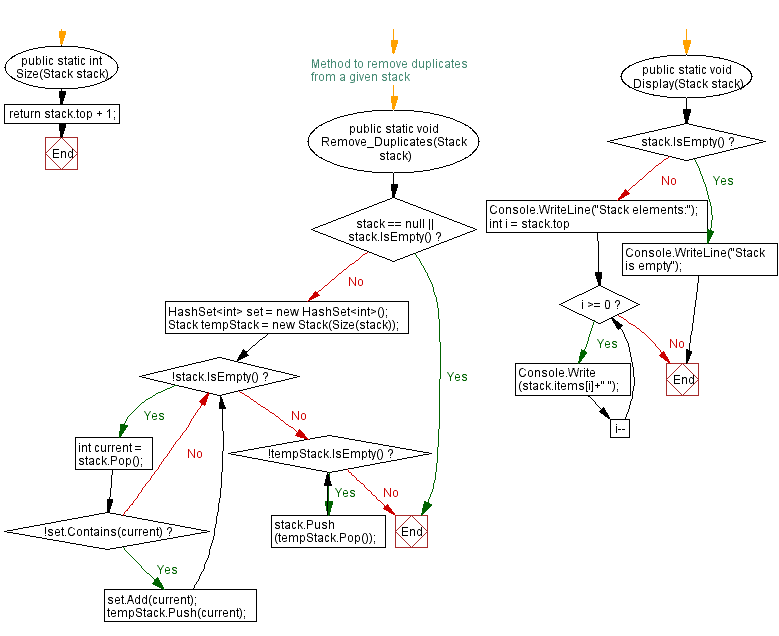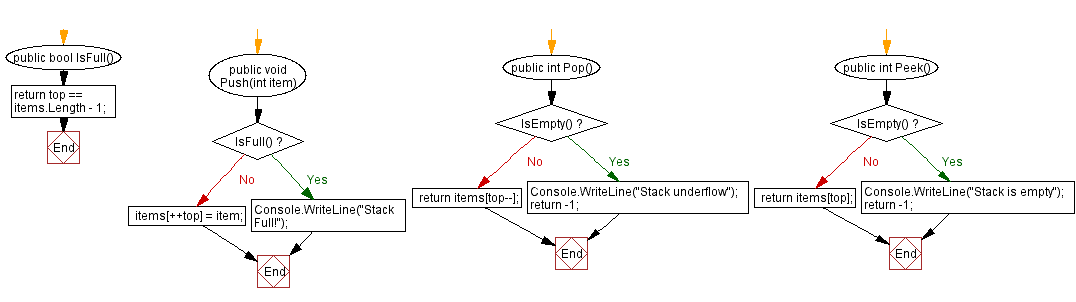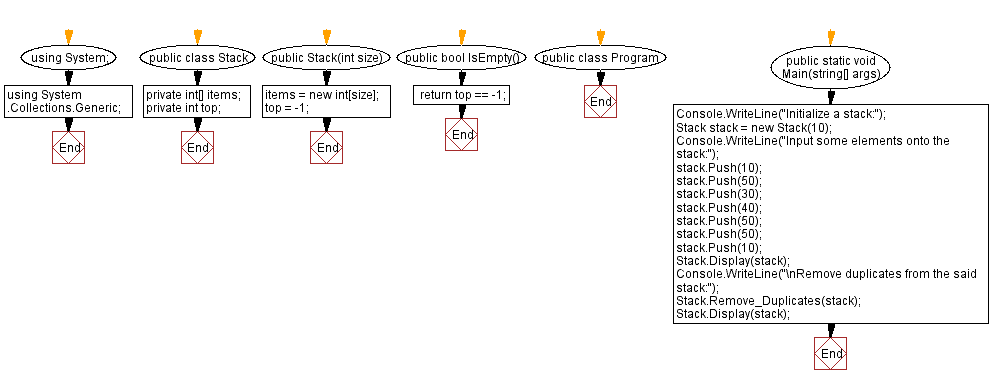﻿ C# - Remove duplicates from a given stack

# C#: Remove duplicates from a given stack

## C# Sharp Stack: Exercise-12 with Solution

Write a C# program to remove duplicates from a given stack.

Sample Solution:

C# Code:

``````using System;
using System.Collections.Generic;
public class Stack
{
private int[] items;
private int top;

public Stack(int size)
{
items = new int[size];
top = -1;
}

public bool IsEmpty()
{
}

public bool IsFull()
{
}

public void Push(int item)
{
if (IsFull())
{
Console.WriteLine("Stack Full!");
return;
}

items[++top] = item;
}

public int Pop()
{
if (IsEmpty())
{
Console.WriteLine("Stack underflow");
return -1;
}

return items[top--];
}

public int Peek()
{
if (IsEmpty())
{
Console.WriteLine("Stack is empty");
return -1;
}

return items[top];
}

public static int Size(Stack stack)
{
return stack.top + 1;
}

// Method to remove duplicates from a given stack
public static void Remove_Duplicates(Stack stack)
{
if (stack == null || stack.IsEmpty())
return;

HashSet set = new HashSet();

Stack tempStack = new Stack(Size(stack));

while (!stack.IsEmpty())
{
int current = stack.Pop();
if (!set.Contains(current))
{
tempStack.Push(current);
}
}

while (!tempStack.IsEmpty())
{
stack.Push(tempStack.Pop());
}
}

public static void Display(Stack stack)
{
if (stack.IsEmpty())
{
Console.WriteLine("Stack is empty");
return;
}

Console.WriteLine("Stack elements:");
for (int i = stack.top; i >= 0; i--)
{
Console.Write(stack.items[i]+" ");
}
}

}

public class Program
{
public static void Main(string[] args)
{
Console.WriteLine("Initialize a stack:");
Stack stack = new Stack(10);
Console.WriteLine("Input some elements onto the stack:");
stack.Push(10);
stack.Push(50);
stack.Push(30);
stack.Push(40);
stack.Push(50);
stack.Push(50);
stack.Push(10);
Stack.Display(stack);
Console.WriteLine("\nRemove duplicates from the said stack:");
Stack.Remove_Duplicates(stack);
Stack.Display(stack);
}
}
``````

Sample Output:

```Initialize a stack:
Input some elements onto the stack:
Stack elements:
10 50 50 40 30 50 10
Remove duplicates from the said stack:
Stack elements:
10 50 40 30
```

Flowchart:C# Sharp Code Editor:

Improve this sample solution and post your code through Disqus

What is the difficulty level of this exercise?

Test your Programming skills with w3resource's quiz.

﻿